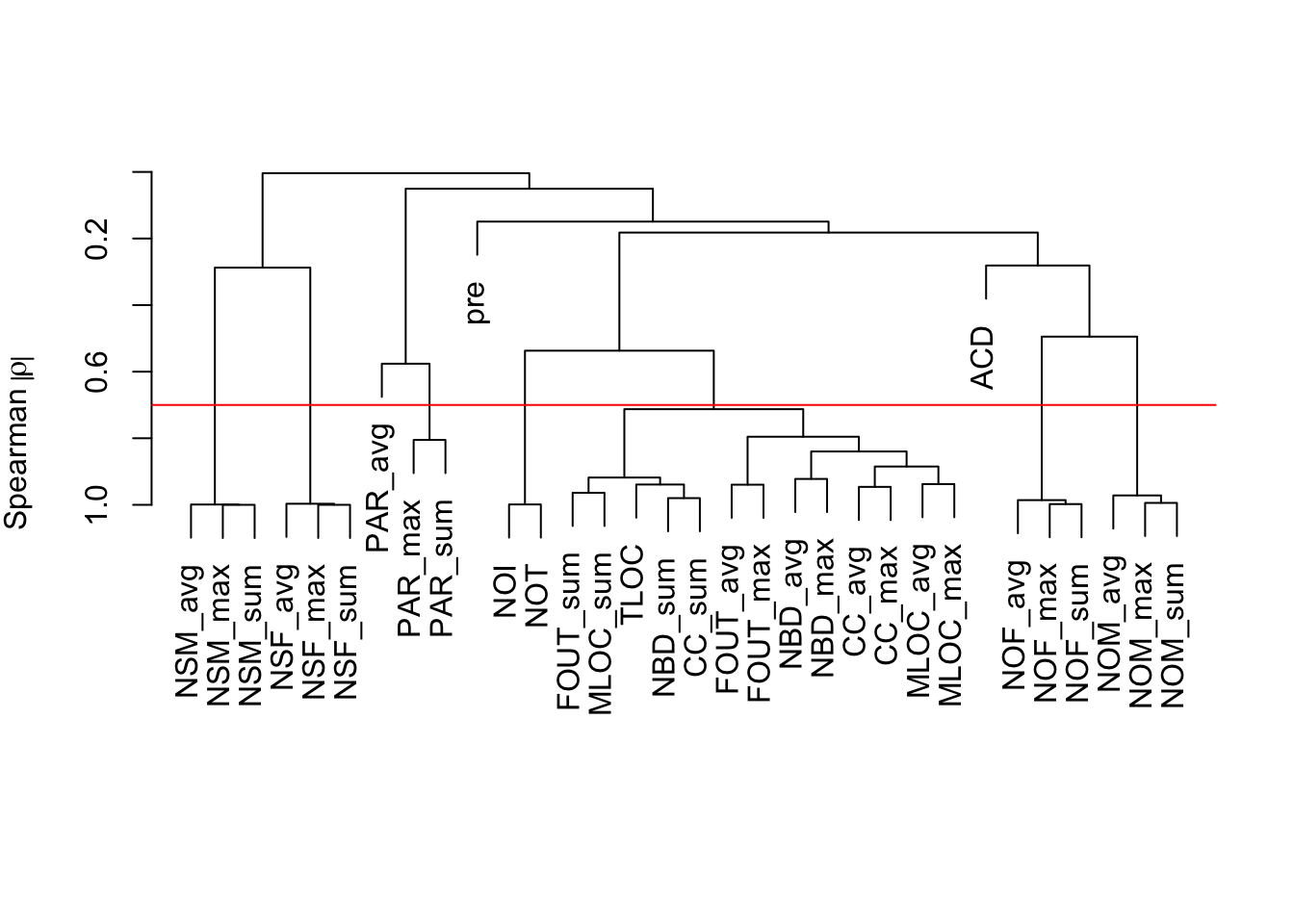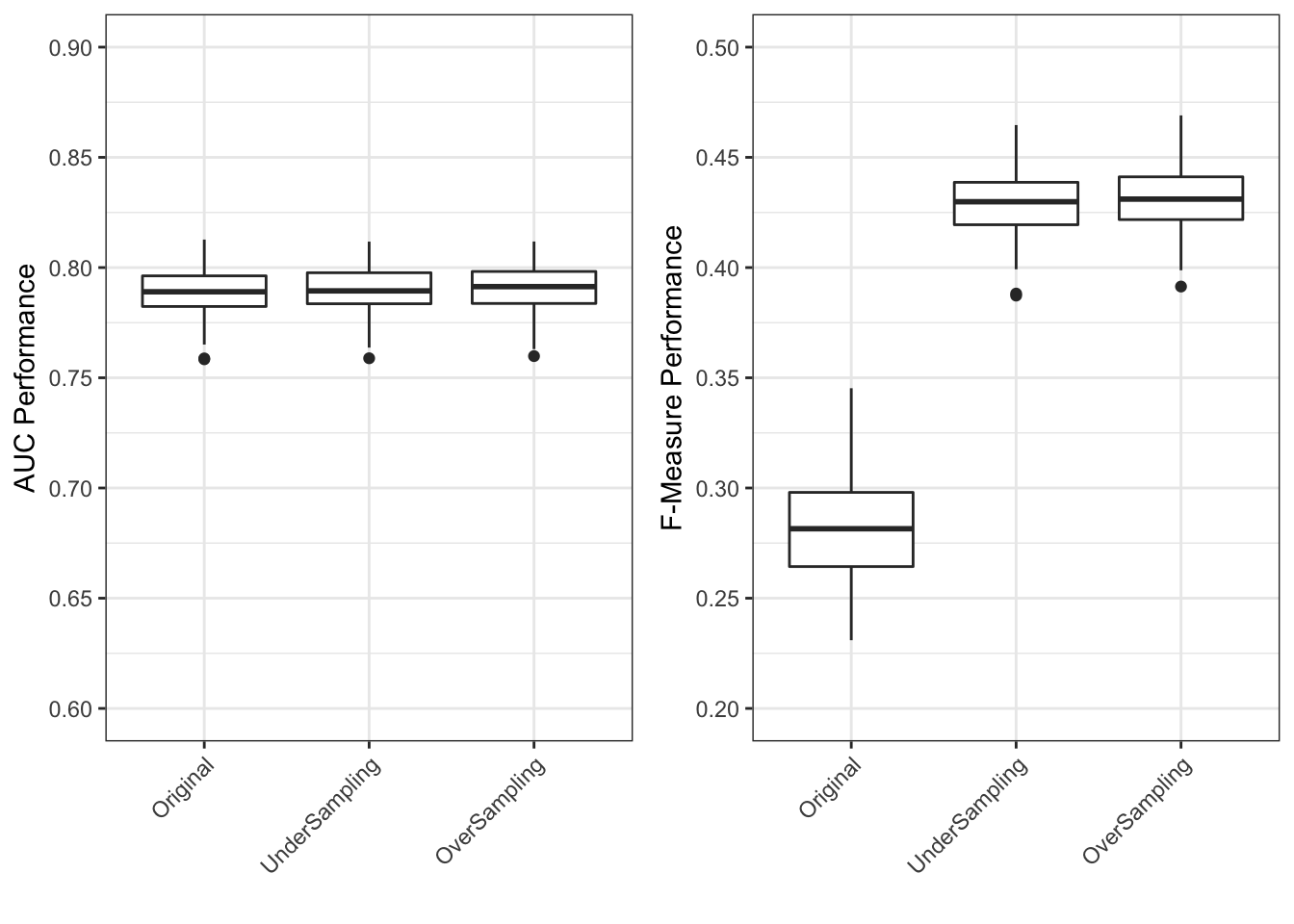Abstract. Over the past decade with the rise of the Mining Software Repositories (MSR) field, the modelling of defects for large and long-lived systems has become one of the most applications of MSR. The findings and approaches of such studies have even attracted the attention of many of our industrial collaborators (and other practitioners worldwide). At the core of many of these studies is the development and use of analytical models for defects. In this paper, we discuss common misconceptions and pitfalls that we observed as practitioners attempt to adopt such models or reason about the findings of such studies. The key goal of this paper is to document such misconceptions and pitfalls so practitioners can avoid them in future efforts. We also hope that other academics will be mindful of such misconceptions and pitfalls in their own work and industrial engagements.

Reproducibility. We provide an access to our experimental data and scripts, which is generated from R markdown using `knitr` R package. This means that all the results from our paper can be reproduced with the code and data available online. This R markdown file can be downloaded at http://chakkrit.com/replication/pitfalls/pitfalls.Rmd

Reference Chakkrit Tantithamthavorn and Ahmed E. Hassan, “An Experience Report on Defect Modelling in Practice: Pitfalls and Challenges”, In Proceedings of 40th International Conference on Software Engineering: Software Engineering in Practice Track (ICSE-SEIP’18), 10 pages.

# Install and load the Rnalytica R package

``````devtools::install_github('software-analytics/Rnalytica')
library(Rnalytica)
library(gridExtra)``````

# Load a running-example defect dataset (Eclipse 2.0)

``````eclipse <- loadDefectDataset("eclipse-2.0")
data <- eclipse\$data
indep <- eclipse\$indep
dep <- eclipse\$dep``````

# Pitfall 1–Testing hypotheses without including control metrics (Table 2)

``````m1 <- fit(data, dep, c("CC_max","PAR_max","FOUT_max"), classifier="lr", validation="boot")
m2 <- fit(data, dep, c("TLOC","CC_max","PAR_max","FOUT_max"), classifier="lr", validation="boot")

c(mean(m1\$performance\$AUC),mean(m2\$performance\$AUC))``````
``##  0.7796273 0.7861877``
``````importance <- data.frame(m1=c(0,anova(m1\$full.model)\$Deviance[-1]), m2=anova(m2\$full.model)\$Deviance[-1])
importance <- data.frame(apply(importance, 2, function(x){x/sum(abs(x))}))
rownames(importance) <- c("TLOC","CC_max","PAR_max","FOUT_max")
round(importance,digit=2)*100``````
``````##          m1 m2
## TLOC      0 82
## CC_max   76  9
## PAR_max  17  7
## FOUT_max  8  2``````

# Pitfall 2–Failure to deal with correlated metrics when interpreting models (Figure 3, Table 3)

``````plot(varclus(as.matrix(data[,indep]), similarity="spear", trans="abs"))
abline(h=0.3, col="red")````````````m1 <- fit(data, dep, c("CC_max","CC_avg","PAR_max","FOUT_max"), classifier="lr", validation="boot")
m2 <- fit(data, dep, c("CC_avg","CC_max","PAR_max","FOUT_max"), classifier="lr", validation="boot")

c(mean(m1\$performance\$AUC),mean(m2\$performance\$AUC))``````
``##  0.7786943 0.7786943``
``````importance <- data.frame(m1=anova(m1\$full.model)\$Deviance[c(3,2,4,5)], m2=anova(m2\$full.model)\$Deviance[-1])
importance <- data.frame(apply(importance, 2, function(x){x/sum(abs(x))}))
rownames(importance) <- c("CC_avg","CC_max","PAR_max","FOUT_max")
round(importance,digit=2)*100``````
``````##          m1 m2
## CC_avg    2 58
## CC_max   74 19
## PAR_max  16 16
## FOUT_max  7  7``````

# Pitfall 3–Class rebalancing techniques improve model performance (Figures 4a and 4b)

``````var <- c("TLOC","PAR_max",'NOI',"NOF_max","FOUT_max","NSM_max","NSF_max","ACD","NOM_max")
original.m <- fit(data, dep, var)

# Check multi-collinearity
vif(original.m\$full.model)``````
``````##     TLOC  PAR_max      NOI  NOF_max FOUT_max  NSM_max  NSF_max      ACD
## 4.326868 1.175210 1.053567 1.866954 1.904298 1.631064 1.572729 1.471195
##  NOM_max
## 2.488857``````
``````down.m <- fit(data, dep, var, classifier="lr", rebalance="down", validation="boot")
up.m <- fit(data, dep, var, classifier="lr", rebalance="up", validation="boot")

auc <- data.frame(Original=original.m\$performance\$AUC,
UnderSampling=down.m\$performance\$AUC,
OverSampling=up.m\$performance\$AUC)
g1 <- ggplot(melt(auc), aes(x=variable, y=value)) + geom_boxplot() + theme_bw() + ylab("AUC Performance") + xlab("") + scale_y_continuous(breaks=12:20*0.05, limits = c(0.6,0.9)) + theme(axis.text.x = element_text(angle = 45, hjust = 1))

fmeasure <- data.frame(Original=original.m\$performance\$Fmeasure,
UnderSampling=down.m\$performance\$Fmeasure,
OverSampling=up.m\$performance\$Fmeasure)
g2 <- ggplot(melt(fmeasure), aes(x=variable, y=value)) + geom_boxplot() + theme_bw() + ylab("F-Measure Performance") + xlab("") + scale_y_continuous(breaks=4:10*0.05, limits = c(0.2,0.5)) + theme(axis.text.x = element_text(angle = 45, hjust = 1))

grid.arrange(g1,g2, ncol=2)````````````x <- original.m\$importance
v <- melt(x)
v\$rank <- sk_esd(x)\$groups[as.character(v\$variable)]
g1 <- ggplot(v, aes(x=variable, y=value)) + geom_boxplot() + facet_grid(. ~ rank, drop=TRUE, scales="free_x", space="free_x") + ylab("Importance Scores") + xlab("Original") + theme(axis.text.x = element_text(angle = 45, hjust = 1),plot.title = element_text(hjust = 0.5)) + ggtitle("Rank")

x <- down.m\$importance
v <- melt(x)
v\$rank <- sk_esd(x)\$groups[as.character(v\$variable)]
g2 <- ggplot(v, aes(x=variable, y=value)) + geom_boxplot() + facet_grid(. ~ rank, drop=TRUE, scales="free_x", space="free_x") + ylab("Importance Scores") + xlab("Under-sampling") + theme(axis.text.x = element_text(angle = 45, hjust = 1),plot.title = element_text(hjust = 0.5)) + ggtitle("Rank")

x <- up.m\$importance
v <- melt(x)
v\$rank <- sk_esd(x)\$groups[as.character(v\$variable)]
g3 <- ggplot(v, aes(x=variable, y=value)) + geom_boxplot() + facet_grid(. ~ rank, drop=TRUE, scales="free_x", space="free_x") + ylab("Importance Scores") + xlab("Over-sampling") + theme(axis.text.x = element_text(angle = 45, hjust = 1),plot.title = element_text(hjust = 0.5)) + ggtitle("Rank")

grid.arrange(g1,g2,g3, ncol=3)``````